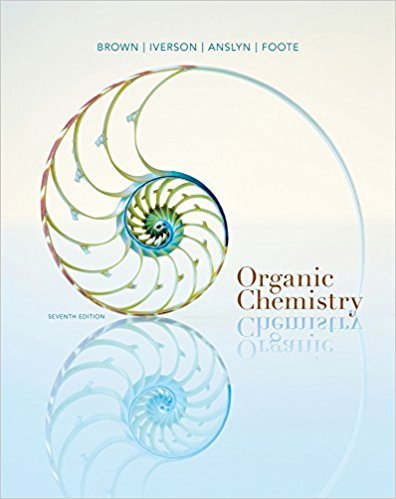×
Get Full Access to Organic Chemistry - 7 Edition - Chapter 22 - Problem 22.35
Get Full Access to Organic Chemistry - 7 Edition - Chapter 22 - Problem 22.35

×

# Show reagents and conditions to bring about the following conversionsISBN: 9781133952848 483

## Solution for problem 22.35 Chapter 22

Organic Chemistry | 7th Edition

• Textbook Solutions
• 2901 Step-by-step solutions solved by professors and subject experts
• Get 24/7 help from StudySoup virtual teaching assistantsOrganic Chemistry | 7th Edition

4 5 1 380 Reviews
30
5
Problem 22.35

Show reagents and conditions to bring about the following conversions.

Step-by-Step Solution:
Step 1 of 3

( Valence electrons are the electrons in the outer orbit) Chemistry chapter 5 G.N. Lewis and Bonding  Lewis theory fundamental ideas: o Valence electrons are the most important in chemical bonding o Two bond type:  Ionic : valence electrons are transferred  Covalent : valence electrons are shared o Bond formation results in formation of full outer Bohr orbits. Because this stable configuration typically involves eight electrons, this is commonly known as the OCTET rule o Noble gasses are already stable Lewis Structure  Element symbol surrounded by a number of dots equal to the number of valence electrons  Ignore the inner or core electrons  The order in which electrons (dots) are drawn and their exact location are not critical Octet and Duet  Elements without an octet tend to react with other elements to form an octet, except hydrogen which reacts to form a duet  Chemical bonding brings together elements in the correct ratios so that all of the atoms involved form an octet Ionic Lewis Structures  Since ionic bonding involves the transfer of electrons from a metal to a nonmetal, the Lewis structure for an ionic compound involves moving dots. The metal becomes a cation and the nonmetal becomes an anion Ionic Lewis Structures  The metal and the nonmetal each acquire a charge in the formation of an ionic bond  We indicate the magnitude of the charges in the upper right corner of

Step 2 of 3

Step 3 of 3

## Discover and learn what students are asking

Calculus: Early Transcendental Functions : Basic Differentiation Rules and Rates of Change
?Finding a Derivative In Exercises 3–24, use the rules of differentiation to find the derivative of the function. $$y=12$$

Calculus: Early Transcendental Functions : Riemann Sums and Definite Integrals
?In Exercises 3–8, evaluate the definite integral by the limit definition. $$\int_{2}^{6} 8 d x$$

Chemistry: The Central Science : The Chemistry of Life: Organic and Biological Chemistry
?Identify the carbon atom(s) in the structure shown that has (have) each of the following hybridizations: (a) s p^{3}, (b) sp,

Unlock Textbook Solution